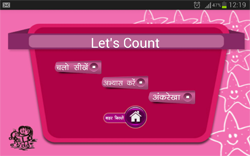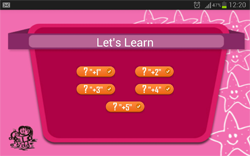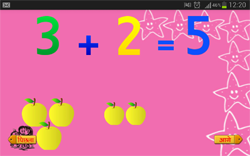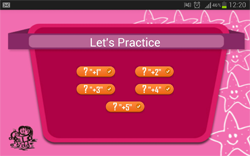## Jumping Numbers AppThis is an app that uses Number line to teach the children the concept of using simple addition facts with cardinal numbers.The app has also got the number bond concepts shown in the most simple way with visual representations, so that it is easier for these children to understand the concept. It also teaches how the numbers are represented using the number line.Number line is a tool which helps children develop greater flexibility in mental arithmetic, as they actively construct mathematical meaning, number sense and the understanding of number relationships. This is also a kind of manipulative used for making the children understand about the number sense and to understand about addition facts of single numbers.

#### Levels in the game

Let's learn

In this level, the number bonds are given for each single number separately with the visual representations. The number bonds go upto number 5 like 1+1=2, 1+2= 3 and so on.

Let's count

The numbers bonds that were taught in the previous level are now given as questions without the visual representation. And the user has to choose the right answer from a set of options given below each sum.

Number line

A predefined number line is given with the numbers marked in that, using that the user has to calculate the given simple addition sums.

## Thinking mathematically using number line concept

Why use number line for mathematical operations?

Number line is a very useful tool for children to practice counting with ordinal numbers and to use it for some basic mathematical operations like addition and subtraction.

It is a very good tool to help children develop greater flexibility in mental arithmetic, number sense and in understanding of number relationships.

It is a type of manipulative used to represent numbers and number operations. It has great many advantages in helping the child understand the relative magnitude and position of numbers as well as to visualize the operations.

It is easier for young children to recognize marks on a number line as visual representations of numbers.

The open line allows the children to use the same tool to understand the variety of number representations, like from 0 to10 or from 20 to 30 and so on. There is also a lot of flexibility in counting strategies that can be used on the number line like from counting by ones to counting by 2's and tens and hundreds.

Using numberline for mathematical operations helps them to be more cognitively active, it is better than visualisation of stationary groups of objects.

When the children use their fingers to jump along the number line, it gives them the feeling of physically counting the numbers.

When they are moving along the number line, they are able to keep a better track of the steps that they are taking, leading to a decrease in the memory load which is necessary to solve the problem. And since the user has moved forward in the number line they will not get the doubt of leaving out some number while counting.